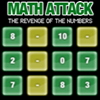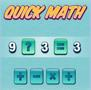Custom Search

# Find Our Cool Math Games to Play by Category:

### 3.OA.B.5

Apply properties of operations as strategies to multiply and divide.2 Examples: If 6 × 4 = 24 is known, then 4 × 6 = 24 is also known. (Commutative property of multiplication.) 3 × 5 × 2 can be found by 3 × 5 = 15, then 15 × 2 = 30, or by 5 × 2 = 10, then 3 × 10 = 30. (Associative property of multiplication.) Knowing that 8 × 5 = 40 and 8 × 2 = 16, one can find 8 × 7 as 8 × (5 + 2) = (8 × 5) + (8 × 2) = 40 + 16 = 56. (Distributive property.)CCSS: 3.OA.B.5
Clear all of the pieces as quickly as you can by building valid equations from the pieces.CCSS: 3.OA.B.5
Choose the right operation for each equation as quickly as you can for a high score. Rating: “Q” for quick!